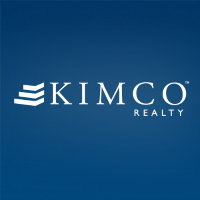# Kimco Realty Corp NYSE:KIMKimco Realty Corp
NYSE:KIM
Price: 19.93 USD +3.16%
Updated:

## DCF Value

This DCF valuation model was created byAlpha Spread and was last updated on Dec 1, 2023.

Estimated DCF Value of oneKIM stock is 9.62 USD. Compared to the current market price of 19.93 USD, the stock is Overvalued by 52%.

DCF Valuation FAQ:
KIM DCF Value
Base Case
9.62 USD
Overvaluation 52%
DCF Value
Price9.62
DCF Value
Kimco Realty Corp Competitors:
DCF Valuation
SPG
Simon Property Group Inc
BRX
Brixmor Property Group Inc
PECO
Phillips Edison & Co Inc
SRC
Spirit Realty Capital Inc
SRG
Seritage Growth Properties

Revenue
1.8B 2B
Net Income
600m 462m

## Present Value Calculation

The first step in the DCF calculation process is to estimate the company's future free cash flow. Once free cash flow is forecasted, it is discounted at a risk-appropriate discount rate. The resulting value is the present value of the company's free cash flow. You can change any model inputs below.

Available Valuation Models
1 valuation model availableUpdated on Dec 1, 2023
Default Valuation
Other investors haven't shared any valuation models for Kimco Realty Corp.
Model Settings
Operating Model
Discount Rate
7.77%
Forecast Period
5 Years
Terminal Growth
0%
Discount Rate
7.77%
Terminal Growth
0%
Growth Period
5 Years

### DCF Model Base Case ScenarioDCF Model
Currency: USD
Millions

To view the process of deriving the DCF Value of one share from the estimated Present Value, see the DCF Value Calculation block.

## DCF Value Calculation

Depending on which type of operating model is chosen (equity or whole firm valuation model), the resulting value is either the value of equity or the value of the entire firm. In the case of the latter, to move from the value of the firm to the value of equity, liabilities are subtracted and assets are added.

### Capital Structure From Present Value to DCF ValuePresent Value 6B USD Equity Value 6B USD / Shares Outstanding 620m KIM DCF Value 9.62 USD
Overvalued by 52%

To view the process of calculating the Present Value of Kimco Realty Corp' future free cash flow, see the Present Value Calculation block.

Discover More

## What is the DCF value of one KIM stock?

Estimated DCF Value of oneKIM stock is 9.62 USD. Compared to the current market price of 19.93 USD, the stock is Overvalued by 52%.

The true DCF Value lies somewhere between the worst-case and best-case scenario values. This is because the future is not predetermined, and the stock's DCF Value is based almost entirely on the future of the company. Knowing the full range of possible stock DCF values gives a complete picture of the investment risks and opportunities.

## How was the DCF Value calculated?

1. Present Value Calculation. Using the DCF Operating Model we projectKimco Realty Corp's future free cash flow and discount it at a selected discount rate to calculate its Present Value (6B USD).

2. DCF Value Calculation. We use the company's capital structure to calculate the total Equity Value based on the previously computed Present Value of the free cash flow. Dividing the Equity Value by the number of shares outstanding gives us the DCF Value of 9.62 USD per oneKIM share.